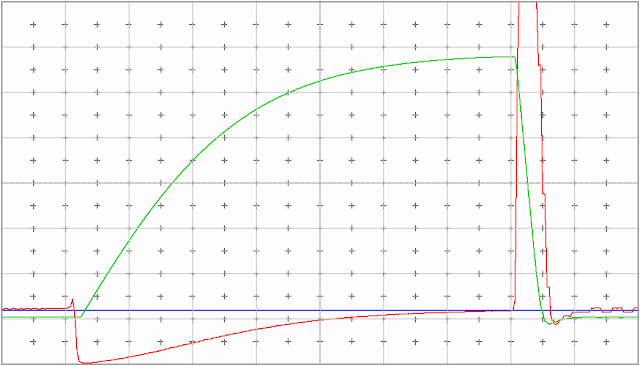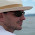## Monday, October 22, 2012

### Modified PID Controller with Constrained Cubic Spline Error Function

A PID controller is a good tool to have in your belt. You can use it as the first approach for most of the control problems a practical electronics engineer faces. You don't have to have the complete dynamic model of your system to be able to use it. What you have to know is how to tune it. After some time you will start feeling how the gains affect the system, and how to test the limits of the system to determine stability.

My  comments here, most likely,  will freak out my  control theory teachers, but what the heck. Sometimes, most of the time to be more precise, you don't have all the tools you need to evaluate your system for several reasons. And most of the time a PID controller will be good enough to move you project forward.

Just to give some context here, I'm not talking about the industrial PID controllers implemented as a box you can buy and connect to your boiler. Here the PID refers to the algorithm and it's implementation as a piece of software.

In this post I will present a modification of the classical PID controller to enable a kind of symmetrical behaviour when the input changes to saturation limits.

This idea occurred to me when I was working with video cameras and having some issues with the auto exposure system. Particularly with the control of the electromechanical iris in the lenses. The control of the iris was achieved by a standard PID, which produced a very noticeable difference in the convergence speed when moving the camera from a bright to a dark scene compared to performing the opposite (dark to bright). This produced one annoying very bright picture that could last for some seconds. I had no luck in tweaking the gains of the controller because while it solved the problem in one condition, it created instabilities in the other.

Analysis of the problem led me to develop the improvement describe here.

### The PID equation

The equation for the PID controller in it's parallel form is:
$u(t)=K_pe(t)+K_i\int_{0}^{t}{e(\tau)}\,{d\tau} + K_d\frac{d}{dt}e(t)$
where:
$$K_p$$: Proportional gain
$$K_i$$: Integral gain
$$K_d$$: Derivative gain
$$e$$: Error
The error is the difference between the set point and the process variable:
$$e=SP-PV$$
$$SP$$: Set Point
$$PV$$: Process variable (input)
I often use this form to directly implement a discrete PID controller.

In a PID controller, the proportional and integral terms contribute to the convergence speed.
The integral term is necessary to offset the error.

### The Problem

For any physical system there will be saturation in every single part. The input will saturate to an maximum and a minimum level. You can design your controller to work with any range of inputs, which is most probably impractical. Or you can artificially limit the input to a certain range. For digital controllers there is a big chance of this saturation being imposed by your A/D converter or other analog signal conditioning circuit. Other good reason to saturate the input is to avoid numerical instabilities problems.

There some classes of systems where, for some reason, you want to limit the convergence speed, or you cannot improve speed without destabilize it.Fig. 1
Now let's consider what happen if a very fast change in the input lead to a saturation. The error will be constant until the accumulated integral part will be enough to offset it. The error derivative is zero at this point, as the error is not changing.

For a quick analysis, lets consider that the integral part is much bigger then the proportional one and dominates the equation. While this situation persists the output will increase, or decrease, at a constant rate. This is represented in the fig 1. The time between 1 and 2.5 seconds the input saturates at its maximum and starting from 3.5 seconds it saturates at its minimum.

Observe that the period of time necessary to recover from  a saturated minimum and the one to recover from a saturated maximum are very different. When it's max saturated it takes 1.5 seconds, and 6 seconds when at minimum. That is 4 times larger. The reason is that our set point is at 1/4 of the maximum value. The Integral term is the dominating factor:

#### Linear Error Function

The error function for the classical PID controller is:

In the Fig. 2 we can see the error 'curve' plotted for 3 different set points in a saturation limited (bounded) system. Observe that if the set point is set to half of the allowed range (0.5 in this case), the error will have the same absolute value, leading to equivalent raising and falling times for the integral, and for the convergence as a consequence.

### Solution

What I propose is to replace the error function in the PID controller by a constrained cubic spline with some special requirements:
• The three points of the curve are: P1=(0,0.5); P2=(PV, 0) and P3=(1, -0.5)
• The curve has to be smooth at P2 and
• The 1st. derivative at P2 should be -1
• The 1st. derivatives should always be negative, i.e. there will be no overshoot
• It has to be simple enough to be computed at runtime
Fig. 3 shows a plot of the proposed function.Fig. 3
In Fig. 3 we can see the same 3 cases depicted previously . But now, regardless of the set point value, the error at both saturation points have the same absolute value: -0.5 and 0.5. This way the system will converge with approximately the same rate in both directions. Notice also that for SP = 0.5 the curve is the same line as in the original error function.

The ideas is to replace the error function by an spline. The proposed solution are a 2 segments cubic splines whose polynomials are:

$e_1(t) = a_{1} + b_{1}PV(t) + c_{1}PV(t)^2 + d_{1}PV(t)^3$
$e_2(t) = a_{2} + b_{2}PV(t) + c_{2}PV(t)^2 + d_{2}PV(t)^3$

Thus, the error function is given by:

$e(t)=\left\{\begin{matrix} e_1(t) & PV(t) \leq SP\\ e_2(t) & PV(t) > SP \end{matrix}\right.$

The coefficient of the polynomials are functions of the Set Point and must be computed each time this value changes. To calculate the coefficients we must solve the spline equations including the proposed constraints.

The equations bellow are a solution for the two segments cubic spline with the constrains presented above.

$f'_{1}(x_{1})=\frac{2}{\frac{x_{2}-x_{1}}{y_{2}-y_{1}}+\frac{x_{1}-x_{0}}{y_{1}-y_{0}}}$
$f'_{1}(x_{0})= \frac{3(y_{1}-y_{0})}{2(x_{1}-x_{0})}-\frac{f'_{1}(x_{1})}{2}$
$f''_{1}(x_{0})=\frac{-2(f'_{1}(x_{1}) + 2 f'_{1}(x_{0}))}{(x_{1} - x_{0})} + \frac{6 (y_{1} - y_{0})}{(x_{1} - x_{0})^2}$
$f''_{1}(x_{1})=\frac{2(2 f'_{1}(x_{1}) + f'_{1}(x_{0}))}{(x_{1} - x_{0})} - \frac{6 (y_{1} - y_{0})}{(x_{1} - x_{0})^2}$
$d_{1} = \frac{f''_{1}(x_{1}) - f''_{1}(x_{0})}{6 (x_{1} - x_{0})}$
$c_{1} = \frac{x_{1} f''_{1}(x_{0}) - x_{0} f''_{1}(x_{1})}{x_{1} - x_{0}}$
$b_{1} = \frac{(y_{1} - y_{0}) - c_{1}(x_{1}^2 - x_{0}^2) - d_{1}( x_{1}^3 - x_{0}^3)}{x_{1} - x_{0}}$
$a_{1} = y_{0} - b_{1} x_{0} - c_{1} x_{0}^2 - d_{1} x_{0}^3$
$f'_{2}(x_{1})=\frac{2}{\frac{x_{2} - x_{1}}{y_{2} - y_{1}} + \frac{x_{1} - x_{0}}{y_{1} - y_{0}}}$
$f'_{2}(x_{2})= \frac{3(y_{2} - y_{1})}{2(x_{2} - x_{1})}-\frac{f'_{2}(x_{1})}{2}$
$f''_{2}(x_{1})=\frac{-2(f'_{2}(x_{2}) + 2 f'_{2}(x_{1}))}{(x_{2}-x_{1})} + \frac{6 (y_{2} - y_{1})}{(x_{2} - x_{1})^2}$
$f''_{2}(x_{2})=\frac{2(2 f'_{2}(x_{2}) + f'_{2}(x_{1}))}{(x_{2} - x_{1})} - \frac{6 (y_{2} - y_{1})}{(x_{2} - x_{1})^2}$
$d_{2} = \frac{f''_{2}(x_{2}) - f''_{2}(x_{1})}{6 (x_{2} - x_{1})}$
$c_{2} = \frac{x_{2} f''_{2}(x_{1}) - x_{1} f''_{2}(x_{2})}{x_{2} - x_{1}}$
$b_{2} = \frac{(y_{2} - y_{1}) - c_{2}(x_{2}^2 - x_{1}^2) - d_{2}( x_{2}^3 - x_{1}^3)}{x_{2} - x_{1}}$
$a_{2} = y_{1} - b_{2} x_{1} - c_{2} x_{1}^2 - d_{2} x_{1}^3$
$x_{0} = 0$
$y_{0} = 0.5$
$x_{1} = SP$
$y_{1} = 0$
$x_{2} = 1$
$y_{2} = -0.5$

To help with the calculation of the polynomials' coefficients I've developed a small Matlab (Octave) program. Bellow there are some results.

SP=0.1250
a1=   0.50000 b1=  -5.50000 c1=   0.00000 d1=  96.00000
a2=   0.13703 b2=  -1.19679 c2=   0.83965 d2=  -0.27988

SP=0.2500
a1=   0.50000 b1=  -2.50000 c1=   0.00000 d1=   8.00000
a2=   0.29630 b2=  -1.38889 c2=   0.88889 d2=  -0.29630

SP=0.5000
a1=   0.50000 b1=  -1.00000 c1=   0.00000 d1=   0.00000
a2=   0.50000 b2=  -1.00000 c2=   0.00000 d2=   0.00000

SP=0.7500
a1=   0.50000 b1=  -0.50000 c1=   0.00000 d1=  -0.29630
a2=  -6.00000 b2=  21.50000 c2= -24.00000 d2=   8.00000

SP=0.8750
a1=   0.50000 b1=  -0.35714 c1=   0.00000 d1=  -0.27988
a2= -91.00000 b2= 282.50000 c2=-288.00000 d2=  96.00000

#### Limitations

When SP is close to the limits (0 or 1)  the derivatives (slope) became very steep and may cause numeric problems. So this method should be used with caution in its extremes.

### Real Application

This modified controlling method was devised when I was developing a digital controller for the auto-exposure system of video surveillance cameras. The objective of this system is to control the brightness of the image being captured by the camera. It has to be able to perform under very extreme light conditions such as direct sunlight and poorly illuminated indoor areas.
There are three parameters to control in the camera in order to regulate the exposure:
• Image sensor gain
• Shutter speed
• Iris opening
Not all the lenses have a controllable Iris, so the system can operate in two different modes depending on the type of lens installed:
• Iris mode - regulates the amount of light entering in the camera
• Shutter mode - regulates the exposure time of each captured frame
I will present some examples of improvements in both modes.

#### Iris Mode

In this mode the dominant parameter to be controlled is the amount of light enters in the camera. This is accomplished by regulating the opening of a mechanical iris embedded in the lens.
The video bellow shows two similar sequences, the first with the normal PID and the second with the modified version. The sequences consist of moving the camera from a dark to a bright scene.

As we can observe on this video, during the first sequence, the camera overshot and got "blind" for a little more than 2 seconds. This effect is due to the very high hysteresis of the electromechanical iris. Next sequence shows a mere half second dark picture. This represents an 8 fold improvement over the original design.
The figures 4 and 5 are the output of a real-time scope that was monitoring the controller operation when the videos were shot. Figure 4 shows the normal PID and figure 5 is the spline error modified. The major horizontal divisions  represent the time in seconds (10 seconds in total). The vertical axis is a interval form -1 to 1, all the variables were normalized to fit this interval.
The traces captured are:
• Blue: Set point (Illumination reference)
• Red: Input (current measured illumination in the image sensor)
• Green: Integral term (normalized to the interval 1, -1)Fig. 4 - Iris control with standard PID errorFig. 5 - Iris controller with modified spline error

#### Shutter Mode

In this mode the amount of light entering in the camera is fixed, what is controlled is the frame's exposure time. The mechanism that allow us to do this is an electronic shutter implemented in the image sensor itself.
The next video is an example of moving from bright to dark. We don't observe visually a so dramatic improvement  as in the previous case. But, as the graphs  bellow shows, the controller took 4 seconds to  converge with the normal PID and 2 seconds with the improved version.

Also is worth mentioning that the shutter model is linear , so we don't observe an overshoot as in the previous case.

Figures 6 and 7 were captured when above video sequences were taken. It must be noticed that the graphs show a little more than the video sequences. The graphs include moving the camera from dark to bright, that is the point where the red line goes up suddenly.Fig. 6 - Shutter control with standard PID errorFig 7 - Shutter control with modified spline error.

1.1.2.